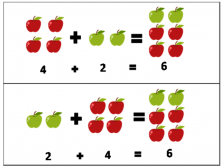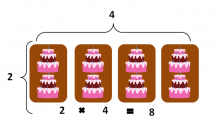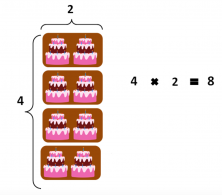Smartick is an online platform for children to master math in only 15 minutes a day

Mar31

# Applying the Commutative Property of Addition and Multiplication in a Problem

Did you know that the commutative property can help us solve an operation faster?  Today we will look at the commutative property of addition and multiplication.

The commutative property tells us that the result of an addition or multiplication is always the same, regardless of the order of the elements with which it operates. Let’s see it in more detail:

## The Commutative Property of Addition

The order of the addends does not change the result
That is, when you have to solve a problem, no matter the order in which you put the addends, you will always get the same result. Why does this happen? Let’s see an example with a problem:

Sara had 4 red apples in her shopping cart. She met Ruth, who gave her 2 green apples. How many apples does Sara have at the end?

To solve this problem, we must add the two types of apples to know how many there are in total. We can add them in two ways:
If we add 4 red apples plus 2 green apples, we get 6 apples in total. Similarly, if we add 2 green apples plus 4 red apples, we also get 6 apples in total.Therefore, no matter the order in which we add the two types of apples, we always get the result of 6 apples in total.

## The Commutative Property of Multiplication

The commutative property of multiplication states:

The order of the factors does not change the product
That is, when we have to solve a multiplication problem, we can arrange the factors in any way we want and always get the same product. Let’s see an example with a problem:

Mark is a baker and today he has received a cake order for a party. They have told him that the party will have 4 tables, and on each of the tables, they want to put 2 cakes. How many cakes will Mark have to make?

To solve this problem we must multiply. We can do this in two ways:If we multiply 2 cakes which will be on each table for four tables, we get 8 cakes.If we multiply 4 tables by 2 cakes in each table, we also get 8 cakes.
Therefore, no matter the order in which you multiply the two numbers, we always get the result of 8 cakes in total.
Whenever you do a problem where you have to multiply or add, remember that you can sort numbers however works best works for you.

What did you think of this post? Do you understand the commutative property better now? If you liked it, share it with your friends so that they can learn too.